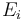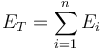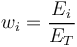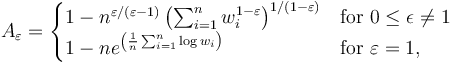# Atkinson Index

## Definition

For the purpose of measuring credit portfolio or market portfolio Concentration Risk, income inequality or diversity, the Atkinson Index is a parametric family of indexes which, given an index parameter epsilon is defined as follows

## Details

If we have n exposures (alternatively values / income measurements)$E_i$ summing up to a total value of$E_T = \sum^{n}_{i=1} E_{i}$

where each observation's fraction is defined as$w_{i} = \frac{E_i}{E_T}$

Then the Atkinson index is given by$A_\varepsilon= \begin{cases} 1- n^{\varepsilon/(\varepsilon-1)} \left(\sum_{i=1}^{n} w_{i}^{1-\varepsilon}\right)^{1/(1-\varepsilon)} & \mbox{for}\ 0 \leq \epsilon \neq 1 \\ 1- n e^{\left(\frac{1}{n} \sum_{i=1}^{n} \log w_{i}\right)} & \mbox{for}\ \varepsilon=1, \end{cases}$
• The index parameter epsilon can take any positive value
• The index varies between zero (homogeneous observations) and one (perfect concentration)

None

None

None

## Implementations

Open Source implementations of the Atkinson index are available in